PTC Thermistors: Definition & Function Thermistors are temperature dependent resistors and "PTC" stands for Positive Temperature Coefficient of resistance. Ceramic PTC thermistor exhibits a highly positive temperature coefficient (Figure 1) where its resistance increases rapidly past a reference temperature (or Curie temperature). The PTC thermistors we produce are based on doped semi- conductive BaTiO3 ceramics.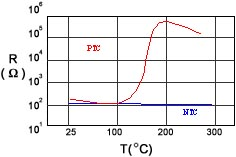FIGURE 1:PTC and NTC thermistor resistance-temperature (R/T) profiles

 In general, applications using PTC thermistor can be categorized according to three unique behaviors: resistance-temperature (R/T), current-voltage (I/V), and current-time (I-t) behaviors. Table 1 gives generalized plots describing these behaviors and their related applications. In many cases, all three behaviors are considered during the design phase.Typical Plots
Function
Applications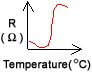R/T
 ˇ´ Sensors ˇ´ Temp controls ˇ´ Over-temp protections ˇ´ Over-current protections ˇ´ Self-regulating heating ˇ´ Degaussing ˇ´ Motor starting ˇ´ Time delay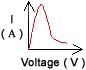I/V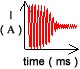I/t

 Key points to consider when using PTC thermistors :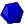PTC performance is a function of heat dissipation conditions, thermistor dimension, Curie temperature, thermistor resistance, applied voltage, etc.PTC thermistor auto-regulates its electrical power in response to thermal dissipations (e.g. a general cooling will "prompt" thermistors to consume more electrical power to maintain its preset temperature).Advantages of PTC thermistor :Insensitivity to voltage fluctuations.Broad operating voltage range (3V to 230V).No controls for temperature or power needed.Self-setting after recovery from faults.Extended electrical & thermal stability Does not glow when energized (no oxygen consumption).Poses no fire hazards.Suitable for wide range of applications.

ABBREVIATED LETTER CODES
 VR =Rated voltage VD =Breakdown voltage VW/S =Withstanding voltage VMAX =Maximum voltage IR =Rated (non-action) current (below this current the PTC thermistor remains in low-resistance state) IS =Switching (action/trip) current (above this current the PTC goes into high-resistance state) IRES =Residual current (corresponding current at steady state applied operating voltage) IMAX =Maximum current IP-P =Peak to peak current value R25C =Resistance (measured @ 25˘J ambient) RMIN =Minimum resistance Ł\ =Temperature coefficient on a R/T curve Ł_ =Dissipation factor (mW/˘J) TSURF =Thermistor surface temperature TOP =Operating temperature PW =Power consumption (electrical wattage measured at a particular) PR =Rated power PG =Gauge power TSW =Switching temperature (temperature corresponding to the resistance which is 2 ˇŃ RMIN) Tr =Reference temperature ŁnOP =Operating time (time period before thermistor switch from low to high resistance state) ŁnR =Recovery time (total time required for thermistor to return to its non-action state) ŁnH =Thermal reaction time ŁX =Thermistor disk diameter ŁXO =Thermistor outer ring diameter ŁXI =Thermistor inner ring diameter DTR =Detective Temperature range